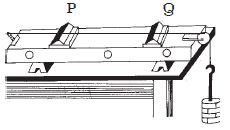# Describe on Sonometer

The sonometer consists of a hollow sounding box about a metre long. One end of a thin metallic wire of uniform cross-section is fixed to a hook and the other end is passed over a pulley and attached to a weight hanger as shown in Figure.The wire is stretched over two knife edges P and Q by adding sufficient weights on the hanger. The distance between the two knife edges can be adjusted to change the vibrating length of the wire.

A transverse stationary wave is set up in the wire. Since the ends are fixed, nodes are formed at P and Q and antinode is formed in the middle.

The length of the vibrating segment is l = λ/

λ = 2l. If n is the frequency of vibrating segment, then

n = v/λ = 2l

We know that v = √(T/m), where T is the tension and m is the mass per unit length of the wire.

n = (1/2l)√(T/m)